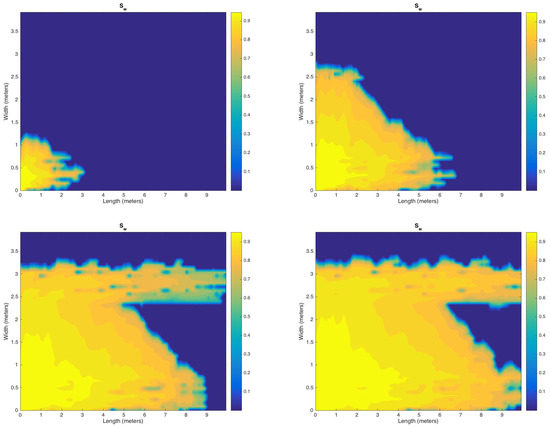Previous Article in Journal
Time-Universal Data Compression

## Volume 12, Issue 6

Article MenuMetrics 0

## Export Article

Open AccessArticle

# Iterative Numerical Scheme for Non-Isothermal Two-Phase Flow in Heterogeneous Porous Media

1
College of Engineering, Effat University, Jeddah 21478, Saudi Arabia
2
Department of Mathematics, Faculty of Science, Aswan University, Aswan 81528, Egypt
Algorithms 2019, 12(6), 117; https://doi.org/10.3390/a12060117
Received: 14 April 2019 / Revised: 22 May 2019 / Accepted: 28 May 2019 / Published: 6 June 2019
|
PDF [1555 KB, uploaded 6 June 2019]
|

# Abstract

In the current paper, an iterative algorithm is developed to simulate the problem of two-phase flow with heat transfer in porous media. The convective body force caused by heat transfer is described by Boussinesq approximation throughout with the governing equations, namely, pressure, saturation, and energy. The two coupled equations of pressure and saturation are solved using the implicit pressure-explicit saturation (IMPES) scheme, while the energy equation is treated implicitly, and the scheme is called iterative implicit pressure, explicit saturation, implicit temperature (I-IMPES-IMT). In order to calculate the pressure implicitly, the equations of pressure and saturation are coupled by linearizing the capillary pressure which is a function of saturation. After that, the equation of saturation is solved explicitly. Then, the velocity is computed which is used in the energy equation to calculate the temperature implicitly. The cell-centered finite difference (CCFD) method is utilized for spatial discretization. Furthermore, a relaxation factor along is used with the Courant–Friedrichs–Lewy (CFL) condition. Finally, in order to illustrate the efficiency of the developed algorithm, error estimates for saturation and temperature for different values of time steps and number of iterations are presented. Moreover, numerical examples of different physical scenarios of heterogamous media are presented. View Full-Text
Keywords:
FiguresFigure 1

This is an open access article distributed under the Creative Commons Attribution License which permits unrestricted use, distribution, and reproduction in any medium, provided the original work is properly cited (CC BY 4.0).# Share & Cite This Article

MDPI and ACS Style

El-Amin, M.F. Iterative Numerical Scheme for Non-Isothermal Two-Phase Flow in Heterogeneous Porous Media. Algorithms 2019, 12, 117.

Note that from the first issue of 2016, MDPI journals use article numbers instead of page numbers. See further details here.

1

# Comments

[Return to top]
Algorithms EISSN 1999-4893 Published by MDPI AG, Basel, Switzerland RSS E-Mail Table of Contents Alert
Back to Top